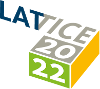#The 39th International Symposium on Lattice Field Theory (Lattice 2022)

Aug 8 – 13, 2022
Hörsaalzentrum Poppelsdorf
Europe/Berlin timezone

## Update on light composite scalar in eight-flavor SU(3) gauge theory

Aug 9, 2022, 8:00 PM
1h
Poster Presentation Particle physics beyond the Standard Model

### Speaker

George Fleming (Yale University)

### Description

We give an update on our ongoing studies of the light composite scalar in eight-flavor SU(3) gauge theory. The chiral limit of this theory can serve as the strong dynamics input to a number of composite Higgs models. Composite Higgs models of this type naturally produce $S$ and $T$ parameters of the size required to explain the new CDF $W$ mass measurement. We present our improved subtraction scheme for scalar correlators at zero spatial momentum. We compare this scheme with results from moving frames at non-zero spatial momentum where no subtraction is required but an assumption must be made regarding the dispersion relation. We also perform an infinite volume extrapolation. Our analysis includes full implementation of the Bayesian model averaging procedure of Jay-Neil which substantially reduces the systematic uncertainties of our previous published results. We will also present first results for the flavor-singlet scalar decay constant and the flavor non-singlet scalar meson mass and decay constant. Finally, we will present two competing EFT analyses: one assuming the light scalar is a pseudo-dilaton and another assuming the massless theory has a strongly-coupled IR fixed point.

### Primary author

George Fleming (Yale University)